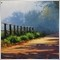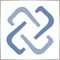# What is wrong with this simple code?53

`#property indicator_chart_window#property indicator_buffers 3#property indicator_plots 3//---- plot high+1/4#property indicator_label1  "high+1/4"#property indicator_type1   DRAW_LINE#property indicator_color1  Red//---- plot midum#property indicator_label2  "midum"#property indicator_type2   DRAW_LINE#property indicator_color2  DeepSkyBlue//---- plot low-1/4#property indicator_label3  "low-1/4"#property indicator_type3   DRAW_LINE#property indicator_color3  DarkSeaGreen//---- buffersdouble HH[];// double MM[];double LL[];//+------------------------------------------------------------------+//| Custom indicator initialization function                         |//+------------------------------------------------------------------+int OnInit()  {//--- indicator buffers mapping   SetIndexBuffer(0,HH,INDICATOR_DATA);   SetIndexBuffer(1,MM,INDICATOR_DATA);   SetIndexBuffer(2,LL,INDICATOR_DATA);//---//--- set accuracy   IndicatorSetInteger(INDICATOR_DIGITS,_Digits);//--- set drawing line empty value   PlotIndexSetDouble(0,PLOT_EMPTY_VALUE,0.0);   PlotIndexSetDouble(1,PLOT_EMPTY_VALUE,0.0);   PlotIndexSetDouble(2,PLOT_EMPTY_VALUE,0.0);      //--- initialization done   return(0);  }int OnCalculate(const int rates_total,                const int prev_calculated,                const datetime& time[],                const double& open[],                const double& high[],                const double& low[],                const double& close[],                const long& tick_volume[],                const long& volume[],                const int& spread[])  {   ArraySetAsSeries(high,true);//  ArraySetAsSeries(close,true);   ArraySetAsSeries(open,true);      int i,limit;    //--- check for rates   if(rates_total<2)      return(0);      //--- preliminary calculations         limit=prev_calculated-1;      //--- the main loop of calculations        for(i=limit;i<rates_total;i++)     {       double h=high[i];     double l=low[i];         double mid=(h+l)/2;     double range=(h-l)/4;              HH[i]=h+range;      MM[i]=mid;      LL[i]=l-range;          }   return(rates_total);  }//+------------------------------------------------------------------+`1496

limit will be -1 if prev_calculated is 053

mql5:

limit will be -1 if prev_calculated is 0

Thanks.

I was confused by pre_calculated.

It seems prev_calculated frequently set Zero,right?53

As per the statement about OnCalculate() in the manual MQL5 as below:

"

We should noted the connection between the return value of OnCalculate() and the second input parameter prev_calculated. During the function call, the prev_calculated parameter contains a value returned by OnCalculate() during previous call. This allows for economical algorithms for calculating the custom indicator in order to avoid repeated calculations for those bars that haven't changed since the previous run of this function.

For this, it is usually enough to return the value of the rates_total parameter, which contains the number of bars in the current function call. If since the last call of OnCalculate() price data has changed (a deeper history downloaded or history blanks filled), the value of the input parameter prev_calculated will be set to zero by the terminal.

As the pice changed frequently, so paremeterr  pre_calculated is set to zero frequently ?

it will burden the indicator calculation, right ???53

mql5:

limit will be -1 if prev_calculated is 0

Thank you. Now it is OK.

Revised as below:

`//--- check for rates   if(rates_total<2)      return(0);      //--- preliminary calculations      limit = prev_calculated-1;   if (limit<0) limit=0;         `